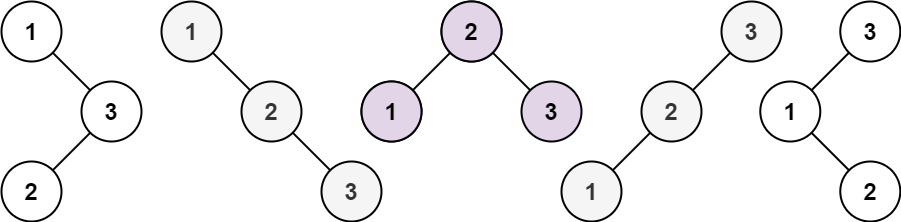# Unique Binary Search Trees (Leetcode 96) Solution - Coding Interview QuestionMani
Educating everyone with the beauty of programming!!

Given an integer `n`, return the number of structurally unique BST's (binary search trees) which has exactly `n` nodes of unique values from `1` to `n`.

Example 1:```Input: n = 3
Output: 5
```

Example 2:

```Input: n = 1
Output: 1
```

Constraints:

• `1 <= n <= 19`

Solution:

``````1
2
3
4
5
6
7
8
9
10
11
12
13
14
15
16
17
18
19
20
21
22
23
24
25
26
27
28
29
30
31
32
33
34
35
36
37
38
39
40
41
42
43
44
45
46
47
48
49
50
51
52
53
54
55
56
57
58
59
60
class Solution {

public int numTrees(int n) {
this.n = n;
this.dp = new int[n + 1][n + 1];
return recurse(1, n);
}

/**
* Intuition: Since we just need to count the number of trees, we can use dp here.
* Number of trees for n i.e. f(n) = total trees with each of the numbers as root nodes from 1 to n.
* For a given node say 2 , total left sub tree * total right sub tree will result in total trees.
* Total = sum[1 to n](recurse(left ) * recurse(right))
**/
public int calculate(int n) {
int[] dp = new int[n + 1];
dp = 1;
dp = 1;

for (int i = 2; i <= n; i++) {
int res = 0;
for (int j = 1; j <= i; j++) {
res += (dp[j - 1] * dp[i - j]);
}
dp[i] = res;
}

return dp[n];
}

int n;
int[][] dp;

/**
* Recursive dynamic programming code. Total = sum[1 to n](recurse(left ) * recurse(right))
* Time Complexity: O(N^2)
* Space Complexity: O(N^2)
*/
public int recurse(int start, int end) {

if (start >= end) {
return 1;
}

System.out.println(start + ":" + end);

if (dp[start][end] != 0) {
return dp[start][end];
}

int res = 0;
for (int i = start; i <= end; i++) {
res += recurse(start, i - 1) * recurse(i + 1, end);
}
dp[start][end] = res;
return res;
}
}
``````
Drop your comments below for any questions or comments. For full list of must learn top interview questions Click Here: Must Learn Top Interview Questions

Rating: